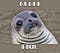# [Python Beginner][DIY] Make Fractal Trees

Before we get into creating a fractal tree, let us understand, how it works.

So a fractal tree works on the principle of Recursion. Basically, recursion is a programming technique that revolves around a function calling itself in iterative steps.

So, how do we do recursion exactly? and why is it being used here?

To do recursion in a function, we must follow 2 simple rules:
1. Make it as logical as possible since debugging a recursion can be a pain
2. Make sure you have a break statement with an if statement inside the recursive function else the function will run till infinity

`def factorial(n):    if n == 1:        return 1    else:        return n * factorial(n-1) # calling the function againprint(factorial(3)) #Output: 6`

In the above example, you can see how recursion is done in python. It is quite straightforward i.e. you call the function again and again till the number n gets reduced to 1 and then it exits the recursion loop.

*Here having the if n==1 statement is extremely important else, recursion will go till negative infinity.

i. The main benefit of a recursive approach to algorithm design is that it allows programmers to take advantage of the repetitive structure present in many problems.
ii. Complex case analysis and nested loops can be avoided.

i. Slowing down execution time and storing on the run-time stack more things than required in a non-recursive approach are major limitations of recursion.
ii. If recursion is too deep, then there is a danger of running out of space on the stack and ultimately program crashes.
iii. Even if some recursive function repeats the computations for some parameters, the run time can be prohibitively long even for very simple cases.Okay….how is all that technical mumbo jumbo related to me making trees?

Well now comes the fun part.

A fractal tree is a tree made up by creating recursive branching in a graphics module in python. We can use turtle module or pygame module.

Since turtle will be easier, we will go with it.

First things first, install turtle module in your python environment. Doing a simple pip install turtle in command line should do the trick.

*For more info on how to do pip install in python, refer here.

Let us do a simple code first before jumping head-first into the fractal tree.

`import turtlemyTurtle = turtle.Turtle() # initialization of the modulemyWin = turtle.Screen() # initialization of the screendef drawSpiral(myTurtle, lineLen):    if lineLen > 0:        myTurtle.forward(lineLen) #move forward        myTurtle.right(90) # move right with a 90 degree angle        drawSpiral(myTurtle,lineLen-5) #draw the above movementsdrawSpiral(myTurtle,100)myWin.exitonclick()`

The above code is pretty straightforward, go forward and then take a right and do it in a loop with reducing distance and voila! spiral.

Now to make a tree.

First, we need to make the branches, the basic idea is to go forward, move right then back to the origin and move left to make a ‘Y’ shape. Repeat it on a loop and pretty much done. Adjust the branch length for making a more complex tree.

`import turtledef tree(branchLen,t):    if branchLen > 5:        t.forward(branchLen)        t.right(20)        tree(branchLen-15,t)        t.left(40)        tree(branchLen-15,t)        t.right(20)        t.backward(branchLen)def main():    t = turtle.Turtle()    myWin = turtle.Screen()    t.left(90)    t.up()    t.backward(100)    t.down()    t.color("green")    tree(75,t)    myWin.exitonclick()main()`

Play around the code a bit and try if you can make an even more complex tree.